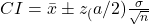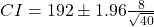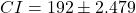## A group of 40 bowlers showed that their average score was 192. Assume the population standard deviation is 8. Find the 95% confidence interv

Question

A group of 40 bowlers showed that their average score was 192. Assume the population standard deviation is 8. Find the 95% confidence interval of the mean score of all bowlers.

in progress 0
3 months 2021-08-06T09:35:22+00:00 1 Answers 7 views 0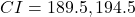Step-by-step explanation:

From the question we are told that:

Sample size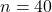Mean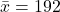Standard deviation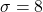Significance Level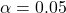From table

Critical Value of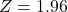Generally the equation for momentum is mathematically given by